# All 64 GMAT Math Formulas You Need + How to Use Them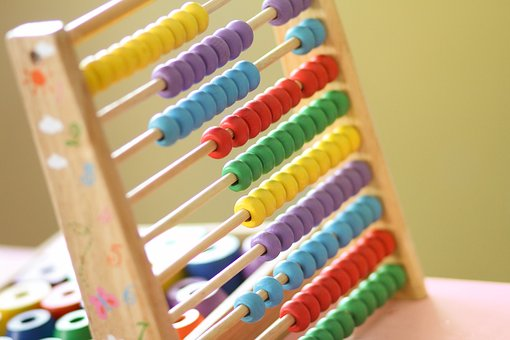Have you ever heard of Dave Hopla? He’s a basketball coach. Well, more specifically, he’s a basketball shooting coach. Throughout his career, he’s made 98 percent of the shots he’s taken. And, he trains players to do the same. How? By mastering the fundamentals.

You might be wondering why I’m beginning an article about the GMAT by talking about a basketball coach. Well, in this article, I’m going to be talking about the importance of mastering GMAT math formulas. Mastering GMAT math formulas is like practicing your free throw if you’re a basketball player. It’s a way to build up your fundamental skills so that, on test day, you’re able to achieve your goal score.

In this article, I’ll talk about why GMAT quant formulas are important, break down the most important formulas to know by subject, and give you some tips for how to incorporate formulas into your prep. I’ll also give you access to a downloadable PDF that you can use to practice offline.

## Why Do You Need to Know Math Formulas for the GMAT?

Spending time learning and memorizing GMAT formulas may seem like an overly simple task in light of the complex questions you’ll face on the GMAT quant section, but studying these formulas is an important part of reaching your goal score.

Despite the notoriety of the GMAT quant section, the questions on this section actually test basic math concepts that are presented in complex ways. Mastering fundamentals, like formulas, will help you solve these questions because you’ll be able to figure out the answers to each individual part of the problem, leading to your final answer.

Not sure how or what to study? Confused by how to improve your score in the shortest time possible? We've created the only Online GMAT Prep Program that identifies your strengths and weaknesses, customizes a study plan, coaches you through lessons and quizzes, and adapts your study plan as you improve.

We believe PrepScholar GMAT is the best GMAT prep program available, especially if you find it hard to organize your study schedule and don't want to spend a ton of money on the other companies' one-size-fits-all study plans.The GMAT quant section only tests math concepts that you would’ve learned in high school. So, learning basic formulas will go a long way in ensuring you understand how to solve different questions.

Learning formulas is also a big time-saver. You only have a limited amount of time for the GMAT quant section, so you’ll need to work quickly and efficiently to answer every question, which is important to do so that you maximize your score.

By learning these formulas, you’ll be able to easily recall what you should do to answer each question. You’ll save time by using the most efficient methods for solving a question.

The GMAT quant section doesn’t allow you to use a calculator. So, you won’t be able to easily do long, complex calculations. Learning formulas will help you because you’ll have tools at your disposal to figure out different measurements or calculations without technology.

While learning formulas is an important part of studying for the GMAT, it’s not all you should do to prepare for the quant section. As I mentioned before, while the actual math on the GMAT is relatively simple, the questions are presented in a complex way. You’ll need to spend time applying your formula knowledge by practicing the different styles of questions on the GMAT. Studying in this way will help you feel prepared for the questions you’ll see on test day.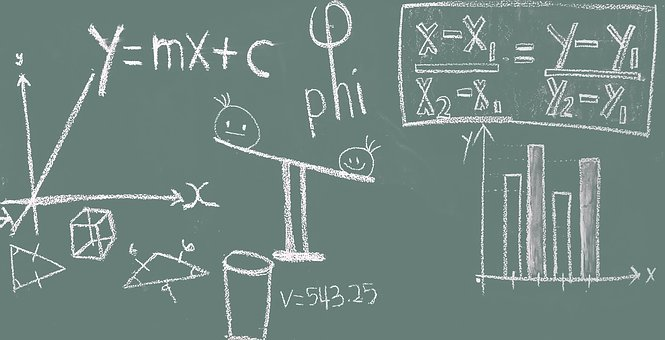## GMAT Quant Formulas

In this section, I’ll break down the most important formulas for you to know for the GMAT. I’ve broken them down by math topic so that they’re easier to sort through.

### GMAT Geometry Formulas

Geometry questions make up about 20% of the questions test on the GMAT quant section. Use these formulas to master the content covered on those questions.

#### AREA & PERIMETER FORMULAS

Square:

• Area = \$\length^2\$
• Perimeter = 4*length

Rectangle:

• Area = length*width
• Perimeter = 2(length) + 2(width)

Parallelogram:

• Area = base*height
• Perimeter = 2(base) + 2(height)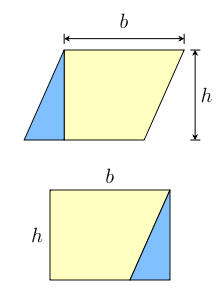Circles:

• Area = \$πr^2\$
• Circumference = \$2πr\$

Triangle:

• Area = \$(\base*\height)/2\$
• Pythagorean Theorem: \$a^2 + b^2 = c^2\$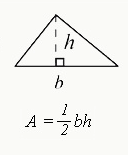Trapezoid:

• Area = \$(1/2)*(a+b)/h\$, where a and b are the length of the parallel sides

#### CIRCLE FORMULAS

Central angle = 2(inscribed angle)

Area of a sector = \$(x/360)*πr^2\$, where \$x \$is the measurement of the central angle of the circle portion in degrees#### VOLUME FORMULAS

Cube: \$(\length)^3\$

Rectangular prism: length*width*height

Cylinder: \$πr^2*h\$

Cone: \$1/3πr^2*h\$

Pyramid: \$1/3(\base \length*\base \width*\height)\$

Sphere: \$4/3πr^3\$

### GMAT Arithmetic Formulas

Arithmetic concepts are one of the most heavily tested content areas on the GMAT quant section, making up nearly 50% of GMAT quant question types. These GMAT quant formulas address the majority of concepts covered on the GMAT.

#### ORDER OF OPERATIONS

Parentheses – Exponents – Multiplication – Division – Addition – Subtraction (PEMDAS)

#### NUMBER PROPERTIES

(Positive Number) * (Positive Number) = (Positive Number)

(Positive Number) * (Negative Number) = (Negative Number)

(Negative Number) * (Negative Number) = (Positive Number)

(Positive Number) / (Positive Number) = (Positive Number)

(Positive Number) / (Negative Number) = (Negative Number)

(Negative Number) / (Negative Number) = (Positive Number)

(Odd Number) + (Odd Number) = (Even Number)

(Odd Number) – (Odd Number) = (Even Number)

(Odd Number) + (Even Number) = (Odd Number)

(Odd Number) – (Even Number) = (Odd Number)

(Even Number) + (Even Number) = (Even Number)

(Even Number) – (Even Number) = (Even Number)

(Odd Number) * (Odd Number) = (Odd Number)

(Odd Number) * (Even Number) = (Even Number)

(Even Number) * (Even Number) = (Even Number)#### PERMUTATIONS AND COMBINATIONS

Permutation formula: \$nPr = n!/(n-r)!\$

Combination formula: \$nCr = n!/((r!)(n-r)!)\$

#### PROBABILITY

Probability = (Number of favorable outcomes) / (Number of all possible outcomes)

Want to improve your GMAT score by 60 points?

We have the industry's leading GMAT prep program. Built by Harvard, MIT, Stanford, and Wharton alumni and GMAT 99th percentile scorers, the program learns your strengths and weaknesses and customizes a curriculum so you get the most effective prep possible.Probability of events A & B happening = (Probability of A) * (Probability of B)

Probability of either event A or B happening = (Probability of A) + (Probability of B)

### GMAT Algebra Formulas

Algebra questions make up about 20% of the question types you’ll see on the GMAT quant section.

#### ABSOLUTE VALUE

|x| depicts absolute value.

|x| = x

|-x| = x

|x| = |-x|

|x| ≥ 0

|x| + |y| ≥ |x+y|

#### EXPONENT Rules

In the expression \$x^n\$, ‘x’ is the base and ‘n’ is the exponent. The way to interpret is that the base ‘x’ gets multiplied ‘n’ times. For example, \$2^3=2*2*2\$.

Some key rules and formulas for exponents:

\$0^n = 0\$

\$1^n = 1\$

\$x^0 = 1\$

\$x^1 = x\$

\$(x)^-n = 1/x^n\$

\$x^m*x^n = x^(m+n)\$

\$x^m/x^n = x^(m-n)\$

\$(x/y)^n = (x^n)/(y^n)\$

\$(x^m)^n = x^(m*n)\$

#### QUADRATIC EQUATIONS

\$ax^2 + bx + c = 0\$

\$x = (-b ∓ √[b^2 – 4ac]) / 2a\$

#### INTEREST

All interest formulas use the following variables: P = starting principle; r = annual interest rate; t = number of years.

Simple Interest = P*r*t

Annual Compound Interest = \$P(1+r)t\$

Compound Interest = P(1 + r/x)^(xt); x = number of times the interest compounds over the year

#### OTHER Algebra Formulas

Distance = Speed * Time

Wage = Rate * Time

## PrepScholar GMAT Formula Sheet

Download our GMAT formula sheet to help you study your GMAT formulas.

## How to Incorporate GMAT Formulas in Your Prep

As I mentioned before, simply memorizing the formulas I gave you above isn’t a good way to prepare for the GMAT quant section. In order to ensure your formula prep really helps your score, you’ll need to apply your formula mastery. Follow these tips to make your formula knowledge work for you.

### #1: Use Flashcards

Flashcards are a great tool for memorizing GMAT math formulas because they’re easy to use and effective. You can quickly review flashcards on your commute or before you go to bed at night or while you’re scarfing down breakfast on the way to class… really, you can review flashcards any time, any place! By spending time memorizing formulas with flashcards, you’ll be able to quickly recall what you’ll need to do to solve a problem.

Flashcards require you to actively engage with the material. For instance, rather than just trying to memorize a list of many different formulas you’ll use on the quant section, you’ll engage with each formula one by one. You’ll be forced to ask yourself whether or not you really know how to use the formula in a quick, concise way.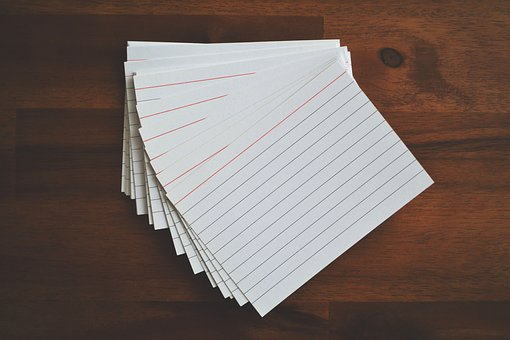### #2: Understand the Underlying Concepts

While memorizing formulas is great, understanding the underlying concepts of each formula is better. Don’t just know what the formulas are – know how they work and, better yet, how to apply them. Understanding the underlying concepts means that you’ve reached a deeper level of learning beyond simply memorizing. If you’re able to understand something, you’ll be able to apply it to solve a question much more easily.

Want to Identify YOUR GMAT Strengths and Weaknesses?

Our proprietary GMAT Diagnostic Assessment creates a customized study plan for you that takes you from registration all the way to test day! It is included with every account and proven to significantly maximize your score.

Get your personalized assessment as part of your 5 day risk-free trial now:Take the formula for area of a sector of a circle: (x/360) * πr2

If you know that a sector is part of a circle that has its own interior angle measurement and you know that the area of a circle is πr2, it makes sense that you can find the area of a sector by multiplying that interior angle by the overall area of the circle.

### #3: Apply GMAT Math Formulas by Practicing Real GMAT Questions

Memorizing formulas won’t help you if you don’t apply your knowledge. The GMAT quant section tests basic concepts in complex ways. You’ll need to spend time familiarizing yourself with the ways that the GMAT asks questions. It won’t simply ask you to find the area of triangle; you might need to find the area of a triangle on your way to discovering how much it costs to put fencing around a triangle-shaped yard.

Spend time learning the style of both problem solving and data sufficiency questions, so that you’ll be able to use your GMAT math formulas to easily get to the correct answer. The best way to practice for the GMAT is to use real retired GMAT questions, which you can find in the official GMAT guides or in the GMATPrep Software. Real, retired GMAT questions best emulate the style and content of what you’ll see on test day.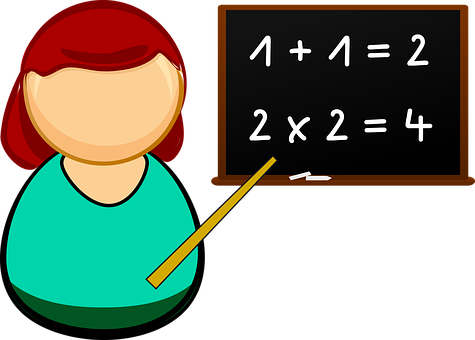## What’s Next?

Looking for a more in-depth review of the quant section? There’s a lot you need to know to be able to reach your GMAT goal score. Our guide to the GMAT quant section teaches you the ten essential tips you need to know to master the most notorious section of the GMAT.

We also offer more specific guides that break down the data sufficiency and problem solving question types on the GMAT. Learn about the types of content covered on the problem solving questions and the trick to mastering the difficult data sufficiency questions, while also learning how to solve sample questions.

Want to focus on a different part of the GMAT? We also offer in-depth guides to the verbal section, as well as tips for how to do well on the analytical writing assessment (link coming soon!). Check out those guides to boost your all-around GMAT scores and get into the business program of your dreams.